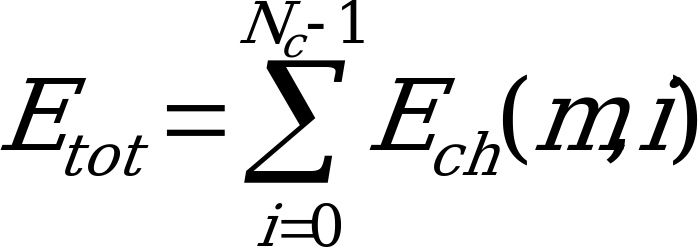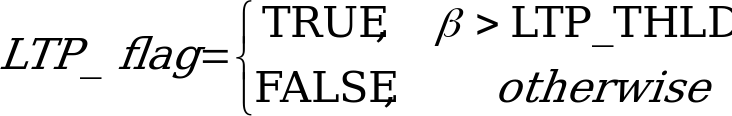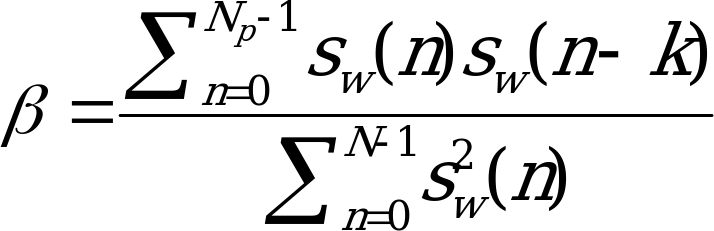## 4.3.10 Background Noise Update Decision

06.943GPPTSVoice Activity Detector (VAD) for Adaptive Multi Rate (AMR) speech traffic channels

The following logic, as shown in pseudo-code, demonstrates how the noise estimate update decision is ultimately made:

/* Normal update logic */
update_flag = fupdate_flag = FALSE
if ( v(m)  UPDATE_THLD and b(m) == 0 ) {
update_flag = TRUE
update_cnt = 0
}

/* Forced update logic (for over-riding the normal update logic)*/
else if (( Etot > NOISE_FLOOR) and ( E(m) < DEV_THLD )
and ( sinewave_flag == FALSE ) and (LTP_flag == FALSE)) {
update_cnt = update_cnt + 1
if ( update_cnt  UPDATE_CNT_THLD )
update_flag = fupdate_flag = TRUE
}

/* "Hysteresis" logic to prevent long-term creeping of update_cnt */

if ( update_cnt == last_update_cnt )
hyster_cnt = hyster_cnt + 1
else
hyster_cnt = 0
last_update_cnt = update_cnt
if ( hyster_cnt > HYSTER_CNT_THLD )
update_cnt = 0

where Etot is the total channel energy defined as:(4.23)

and LTP_flag is generated by the comparison of the long-term prediction gain to a constant threshold LTP_THLD, i.e.:(4.24)

where the long-term prediction gain is derived from the speech encoder open-loop pitch predictor, and can be expressed as:(4.25)

where sw(n) is the weighted speech, k is the optimal open-loop lag, and Np is the pitch analysis frame length. This expression is calculated in the speech encoder on the previous frame.